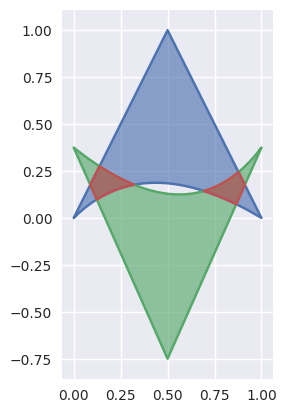# bezier¶

Helper for Bézier Curves, Triangles, and Higher Order Objects

This library provides:

Dive in and take a look!## Why Bézier?¶

A Bézier curve (and surface, etc.) is a parametric curve that uses the Bernstein basis:

$b_{j, n} = \binom{n}{j} s^j (1 - s)^{n - j}$

to define a curve as a linear combination:

$B(s) = \sum_{j = 0}^n b_{j, n} \cdot v_j.$

This comes from the fact that the weights sum to one:

$b_{0, n} + b_{1, n} + \cdots + b_{n, n} = \left(s + (1 - s)\right)^n = 1.$

This can be generalized to higher order by considering three, four, etc. non-negative weights that sum to one (in the above we have the two non-negative weights $$s$$ and $$1 - s$$).

Due to their simple form, Bézier curves:

• can easily model geometric objects as parametric curves, surfaces, etc.
• can be computed in an efficient and numerically stable way via de Casteljau’s algorithm
• can utilize convex optimization techniques for many algorithms (such as curve-curve intersection), since curves (and surfaces, etc.) are convex combinations of the basis

Many applications – as well as the history of their development – are described in “The Bernstein polynomial basis: A centennial retrospective”, for example;

• aids physical analysis using finite element methods (FEM) on isogeometric models by using geometric shape functions called NURBS to represent data
• used in robust control of dynamic systems; utilizes convexity to create a hull of curves

## Installing¶

bezier can be installed with pip:

$python -m pip install --upgrade bezier$ python2.7 -m pip install --upgrade bezier
\$ python3.6 -m pip install --upgrade bezier


bezier is open-source, so you can alternatively grab the source code from GitHub and install from source.

## Getting Started¶

For example, to create a curve:

>>> nodes1 = np.asfortranarray([
...     [0.0, 0.5, 1.0],
...     [0.0, 1.0, 0.0],
... ])
>>> curve1 = bezier.Curve(nodes1, degree=2)


The intersection (points) between two curves can also be determined:

>>> nodes2 = np.asfortranarray([
...     [0.0, 0.25,  0.5, 0.75, 1.0],
...     [0.0, 2.0 , -2.0, 2.0 , 0.0],
... ])
>>> curve2 = bezier.Curve.from_nodes(nodes2)
>>> intersections = curve1.intersect(curve2)
>>> intersections
array([[0.31101776, 0.68898224, 0. , 1. ],
[0.31101776, 0.68898224, 0. , 1. ]])
>>> s_vals = np.asfortranarray(intersections[0, :])
>>> points = curve1.evaluate_multi(s_vals)
>>> points
array([[0.31101776, 0.68898224, 0. , 1. ],
[0.42857143, 0.42857143, 0. , 0. ]])


and then we can plot these curves (along with their intersections):

>>> import matplotlib.pyplot as plt
>>> import seaborn
>>> seaborn.set()
>>>
>>> ax = curve1.plot(num_pts=256)
>>> _ = curve2.plot(num_pts=256, ax=ax)
>>> lines = ax.plot(
...     points[0, :], points[1, :],
...     marker='o', linestyle='None', color='black')
>>> _ = ax.axis('scaled')
>>> _ = ax.set_xlim(-0.125, 1.125)
>>> _ = ax.set_ylim(-0.0625, 0.625)
>>> plt.show()For API-level documentation, check out the Bézier Package documentation.

## Development¶

To work on adding a feature or to run the functional tests, see the DEVELOPMENT doc for more information on how to get started.

bezier is made available under the Apache 2.0 License. For more details, see the LICENSE.